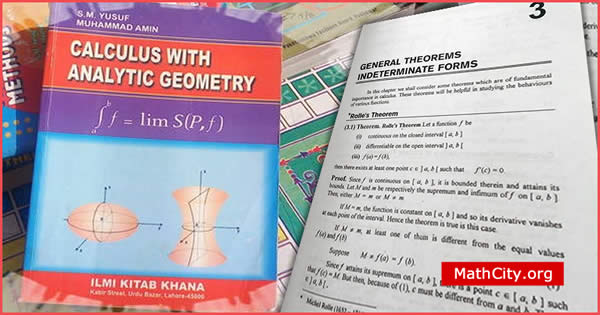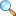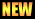• Rolle's theorem
• Geometrical interpretation of Rolle's theorem
• The mean value theorems
• Another form of mean value theorem
• Increasing and decreasing functions
• Cauchy's mean value theorem
• Extended mean value theorem
• Indeterminate form
• The form $\frac{0}{0}$
• The form $\frac{\infty}{\infty}$
• The form $0\times \infty$ (or $\infty \times 0$)
• The form $\infty-\infty$
• The forms $0^\infty, 1^\infty, \infty^0$
• Use of expansions

We are very thankful to Muhammad Idrees for providing notes of Exercise 3.3

• Exercise 3.1 |View Online | Download PDF• Exercise 3.3 |View Online | Download PDF